Curves are fundamental objects in differential geometry. The simplest examples are ones which lie in a plane; curves that we could draw on a piece of paper. We take a look at the Four Vertex Theorem and its Converse, which tell us something about the simple closed curves in the plane.

Curves are fundamental objects in differential geometry. The simplest examples are ones which lie in a plane; curves that we could draw on a piece of paper. In this post, we will look at the Four Vertex Theorem, which is a theorem that applies to a special type of plane curve: the simple closed curve. Simple means that the curve does not cross over itself, and closed means that it must end where it started.

Here are some examples below: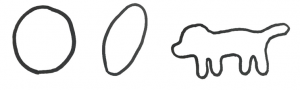One more thing about any curve is that they must be smooth. So, no corners or points, and you must be able to trace it out without lifting pen from paper.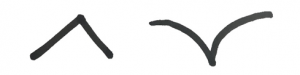Now at any point on a curve we can define curvature; a number that measures how much our curve is curving at that point. If the point is on a straight line, then the curvature would be 0. However, if the curve is turning very quickly, then the points on that turn would have a large curvature. There are also two ways a curve can curve from a to b. One way is assigned a positive number and the other is assigned a negative number.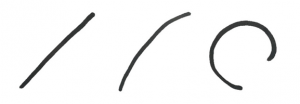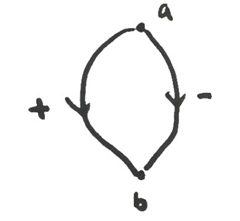So, knowing that we have a simple closed plane curve, is there anything special about the curvature as you go around that curve?

Well the Four Vertex Theorem says yes! Any simple closed curve in the plane must have at least four vertices. Vertices are points on the curve where the curvature is a local maxima or minima. They are points where our curve goes from ‘curving more’ to ‘curving less’, or vice versa. The only exception is the circle, which curves at a constant rate.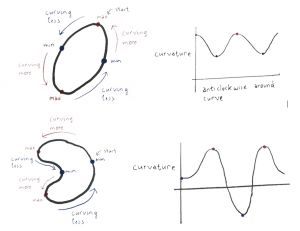What the theorem also tells us is that if your curve does not have four vertices, then it cannot close up.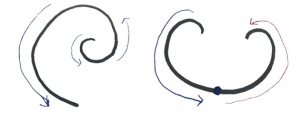Now let’s flip it around. What if we decided the curvature before the curve? Is there a simple closed plane curve out there which has this curvature? As you may expect, there is. The Converse to the Four Vertex Theorem states that as long as your curvature function joins up with itself smoothly, and has at least two local minima and maxima, then we can find a simple closed plane curve for you.

The Four Vertex Theorem and its Converse has been worked on by mathematicians for over a hundred years and is the basis of many generalisations and extensions and even conjectures that we are still unsure of today.

References:

DeTurck, DM, Gluck, H, Pomerleano, D & Shea Vick, D (2007), ‘The four vertex theorem and its converse’, Notices of the American Mathematical Society 54(2), 191–207.

Jia Jia Qian
Monash University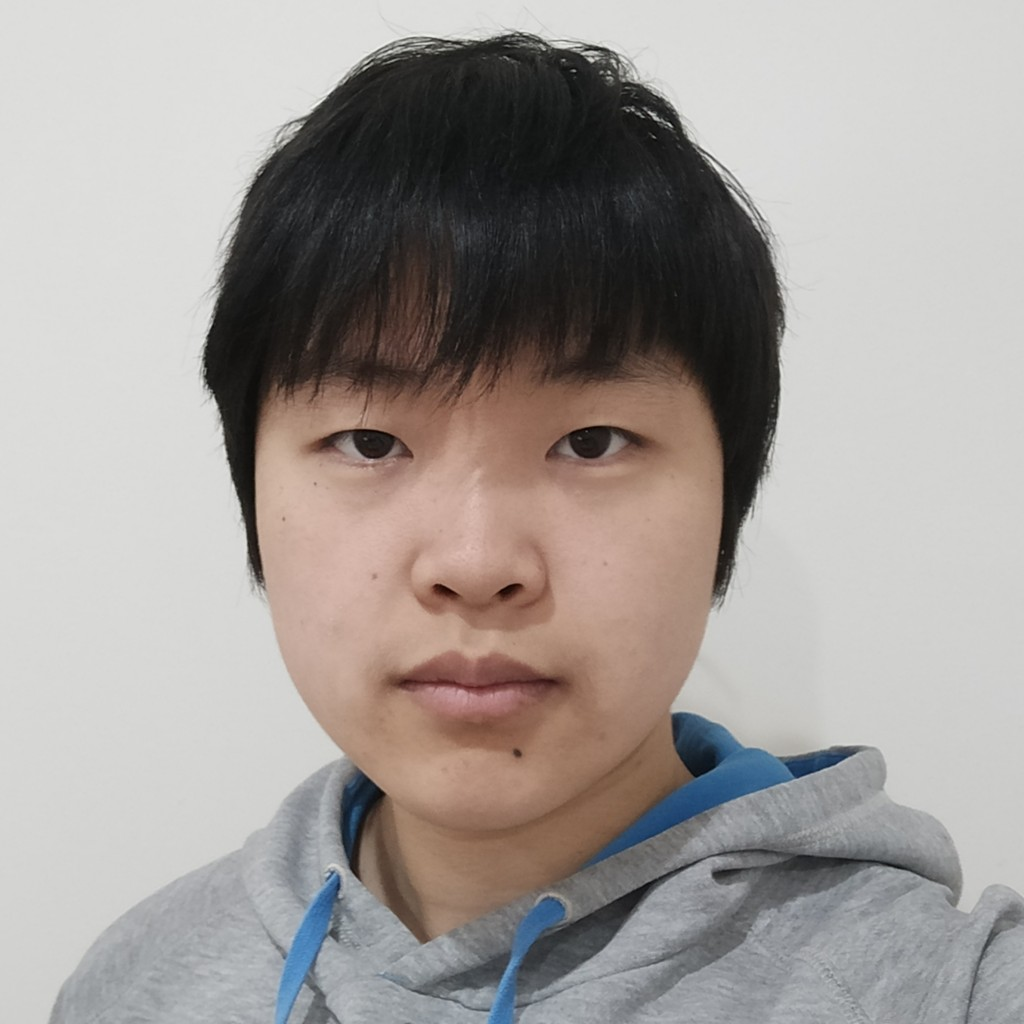View Jia Jia Qian
Profile